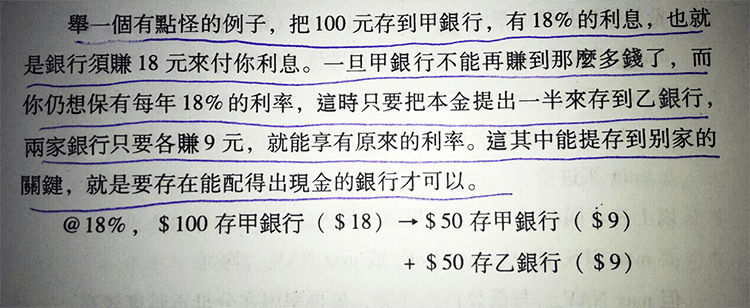# 投資只看ROE會遇上什麼陷阱？

1、如果分紅0元，則期末淨資產=110元，ROE=10/110=9.09%

2、如果分紅5元，則期末淨資產=105元，ROE=10/105=9.52%

3、如果分紅8元，則期末淨資產=102元，ROE=10/102=9.8%

4、如果分紅10元，則期末淨資產=100元，ROE=10/100=10%

1、如果分紅0元，則期末淨資產=110元，淨資產成長率=（110 +0-100）/100=10%

2、如果分紅5元，則期末淨資產=105元，淨資產成長率=（105+5-100）/100=10%

3、如果分紅8元，則期末淨資產=102元，淨資產成長率=（102+8-100）/100=10%

4、如果分紅10元，則期末淨資產=100元，淨資產成長率= （100+10-100）/100=10%

1、如果分紅0元，則期末淨資產=110元，ROE=10/110=9.09%。

2、如果分紅0元，則期末淨資產=140元，ROE=10/140=7.14%。##### 雪球
「雪球」是一個社交投資網絡，它有網頁版（xueqiu.com）和手機客戶端。用戶可以通過雪球：
● 訂閱股票、封基、ETF，全方位收取新聞、公告和用戶討論
● 通過自選股功能查看股票漲跌
● 通過持倉盈虧功能管理個人投資組合
● 和其他投資者實時交流互動